output.to from Sideway
Mechanics: StaticsMechanics Dynamics

2D Plane Body3D Body

Draft for Information Only

# Content

``` First Moment of 2D Plane Body   Centroidal Axis  Symmetry of Plane Body```

# First Moment of 2D Plane Body

The first moment of a two dimensional plane body is defined as the summation of the moments of all elements of the shape of the two dimensional plane body about an axis. The first moment of a two dimensional plane body is the mathematical measure of the distribution of the shape of a two dimensional plane body about an axis. The first moment of a two dimensional plane body can be used to detemine the centroid of the an object. The first moment of a two dimensional plane body is usually called the first moment of area and sometimes is misnamed as the first moment of inertia.In practical problems, the centroid of an plate is determined by the first moment of a two dimensional plane body with the method of the first moment of area.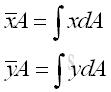And the centroid of a wire is determined by the first moment of a two dimensional plane body with the method of the first moment of line.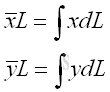The first moment of area can also be used to determine the static moment of area, Q. The static moment of area is a propety to measure the moment of an area about an axis of the shape of a cross-section. The static moment of area is commonly used for determining the distribution of shear stress in flexural members under transverse loadings.## Centroidal Axis

Although the value of first moment of an area is dependent on the reference axes due to the change of reference, the location of the centroid of an area is independent of the reference axes and is the property of the area only.If the first moment of an area about an axis is equal to zero, the centroid of the area must be located on the axis. Conversely, if the centroid of an area is located on an axis, the first moment of the area must be equal to zero also. e.g. the axes x and y have their origin at the centroid.The axes pass through the centroid of an area are called centroidal axes. The first moments of area about any centroidal axis of the area are zero. Since the centroid is located on the centroidal axis, the perpendicular distance from the centroid to the centroidal axis must be zero also.

## Symmetry of Plane Body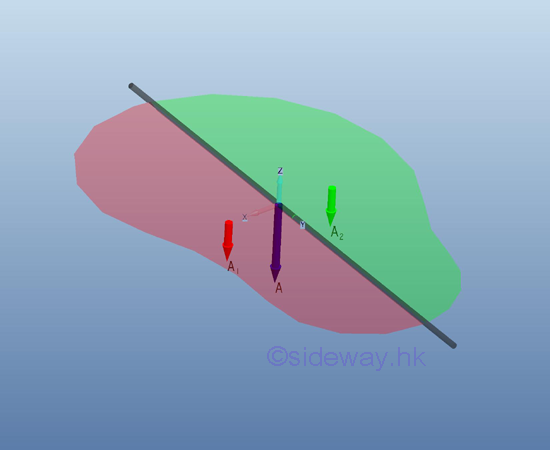A centroidal axis always divide the plane body into two distinct subregions. Since the first moment of area of a plane body about its centroidal axis is equal to zero, the two first moment of area of the two distinct subregions about the centroidal axis of the plane body should be equal and opposite in sense. ImplyConsidering the two subregions of a symmetrical plane body on either side of the axis of symmetry, the elemental areas are equal in size and are equidistant from the axis. For every elemental area on one side of the axis of symmetry, there alway exists an elemental area on the opposite side of the axis of symmetry. And the first moment of these elemental areas will cancel each other because the distances are equal and opposite in sense. Therefore the first moment of a symmetrical plane body about the axis of symmetry is equal to zero and the centroid of the symmetrical plane body should always lies on the symmetric axis also.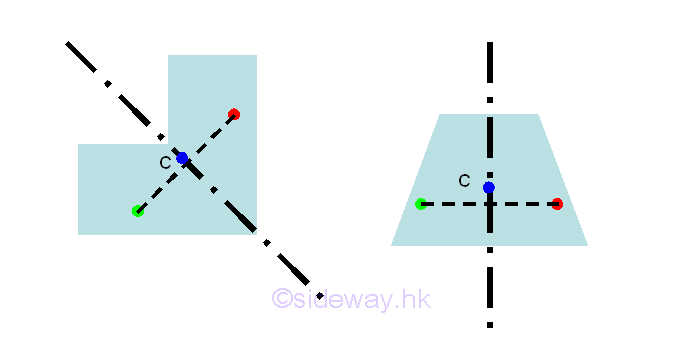Since the centroid of a symmetrical plane body always lies on the symmetric axis, when a plane body possesses two or more axes of symmetry, the centroid of the plane body should always be located at the intersection of the axes.Similarly, the centroid of plane bodies with two or more axes of symmetry can be determined by the construction of axes of symmetry. e.g.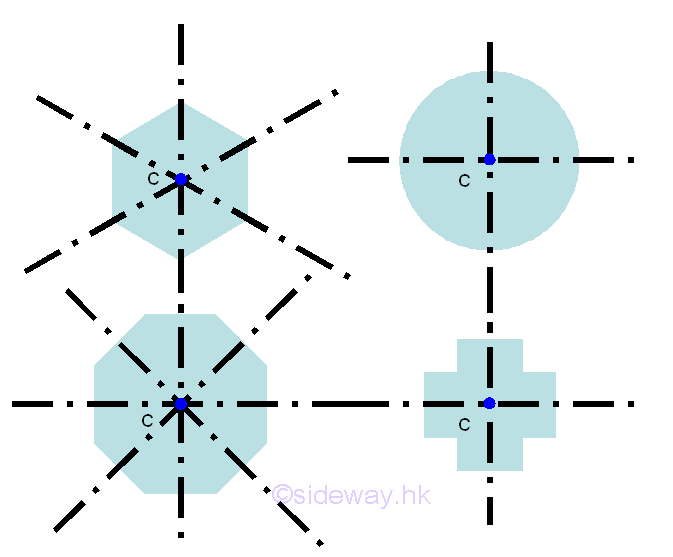Besides the reflection symmetry, a symmetrical plane body can also be rotational symmetric about a point. Geometrically, for every element at coordinate x,y, there always exist an element at coordinate -x,-y. Therefore, a symmetrical plane body with two axes of symmetry at right angle to each other always symmetric about a point. From the above symmetry plane bodies, the centroids of the plane bodys always coincide with the center of symmetry, i.e. intersection of two axes. However, a rotational symmetric plane body sometime does not necessary to be symmetric about an axis. e.g.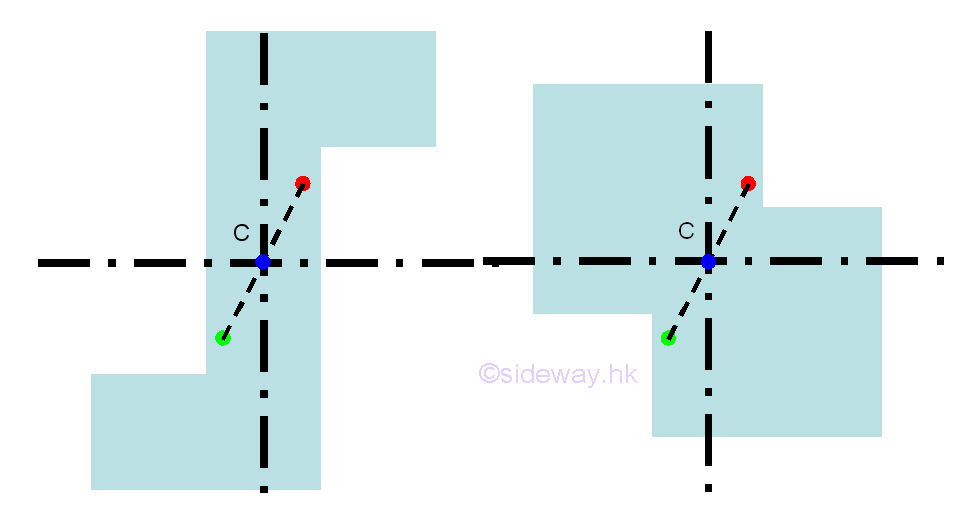ID: 120400003 Last Updated: 13/4/2012 Revision: 0 Ref:References

1. F.P. Beer; E.R. Johnston,Jr.; E.R. Eisenberg, 2004, Vector Mechanics for Engineers: Statics
2. I.C. Jong; B.G. rogers, 1991, Engineering Mechanics: Statics and DynamicsHome 5

Management

HBR 3

Information

Recreation

Culture

Chinese 1097

English 337

Computer

Hardware 151

Software

Application 202

Latex 10

Manim 159

Numeric 19

Programming

Web 285

Unicode 494

HTML 65

CSS 59

ASP.NET 194

OS 391

Python 36

Knowledge

Mathematics

Algebra 29

Geometry 18

Calculus 67

Engineering

Mechanical

Rigid Bodies

Statics 92

Dynamics 37

Control

Natural Sciences

Electric 27